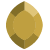# def rungx() makes my script failPosts: 3edited February 23
Hello,

I understand that I need the rungx() if I am running a python script from Oasis. But I don't understand this result:

This code works:
```import geosoft.gxpy as gxpy
import geosoft.gxpy.grid as gxgrd

#def rungx():
#    raise Exception("This is not an Oasis extension.  Please run through a python interpreter.")

gxc = gxpy.gx.GXpy()
xyz=[(450.,10.,100.),(60.,25.,77.),(50.,8.,80.)]
gridName = "test.grd"
grid = gxgrd.Grid.minimum_curvature(xyz,file_name=gridName,overwrite=True)```
while this code fails:
```import geosoft.gxpy as gxpy
import geosoft.gxpy.grid as gxgrd

#def rungx():
#    raise Exception("This is not an Oasis extension.  Please run through a python interpreter.")

gxc = gxpy.gx.GXpy()
xyz=[(450.,10.,100.),(60.,25.,77.),(50.,8.,80.)]
gridName = "test.grd"
grid = gxgrd.Grid.minimum_curvature(xyz,file_name=gridName,overwrite=True)```
So what is going on?

Thanks,
Dave